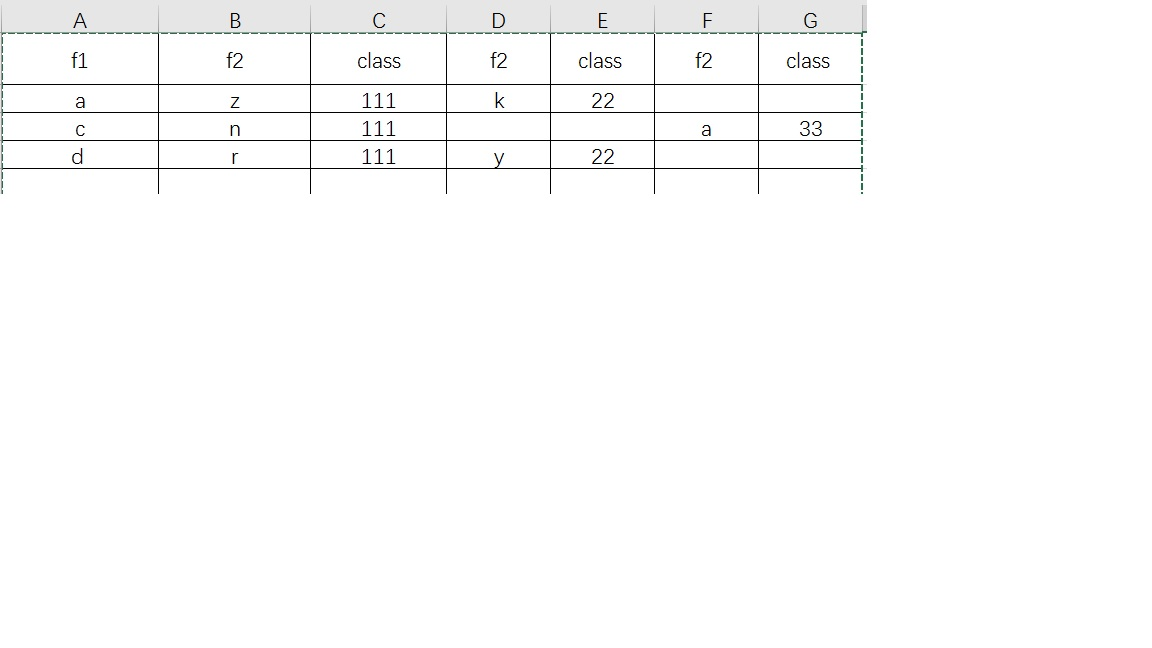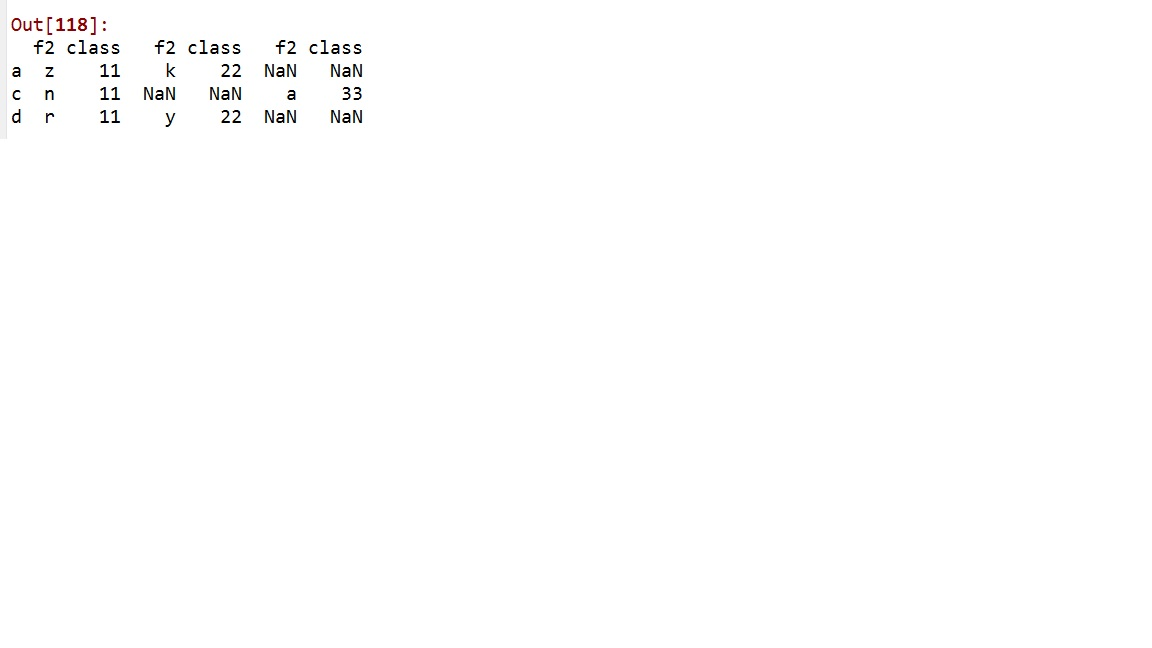###### weixin_40473525

2019-08-06 11:15 阅读 2.2k

# 循环列表中几个小dataframe合并的疑问

[ f1 f2 class
0 a z 11
2 c n 11
3 d r 11, f1 f2 class
1 a k 22
4 d y 22, f1 f2 class
5 c a 33]df5 = pd.concat([df11.set_index('f1'),df22.set_index('f1'),df33.set_index('f1')],axis=1,join='outer')for i in range(len(list2)):

``````c = pd.concat([c, list2[i].set_index('f1')], axis=1, join='outer')
``````

c

• 点赞
• 写回答
• 关注问题
• 收藏
• 复制链接分享

#### 1条回答默认 最新

• pd.concat([**c**, list2[i].set_index('f1')] 这里的c表示的是？

点赞 评论 复制链接分享